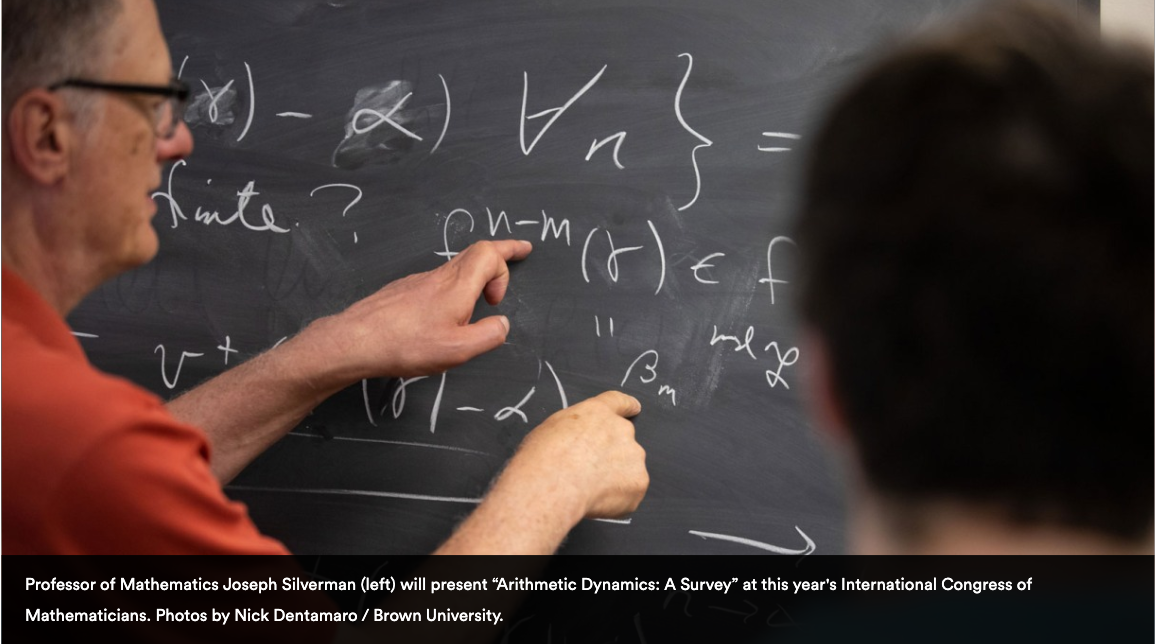•## Welcome to ICERM

### The Institute for Computational and Experimental Research in Mathematics

•## Welcome to ICERM

### The Institute for Computational and Experimental Research in Mathematics

•## Welcome to ICERM

### The Institute for Computational and Experimental Research in Mathematics

•## Welcome to ICERM

### The Institute for Computational and Experimental Research in Mathematics

#### This Week at ICERM

##### March 19, 2023

There are no events currently scheduled for March 19th.

##### March 20, 2023
###### Discrete Optimization: Mathematics, Algorithms, and Computation
• 3:30 - 4:00 pm EDT
Coffee Break
11th Floor Collaborative Space
##### March 21, 2023
###### Discrete Optimization: Mathematics, Algorithms, and Computation
• 9:00 - 10:00 am EDT
Professional Development: Hiring Process
Professional Development - 11th Floor Lecture Hall
• 10:30 - 11:15 am EDT
Memory-efficient approximation algorithm for SDPs and its application to Max-Cut, Max-k-Cut, and Correlation Clustering
Seminar - 11th Floor Lecture Hall
• Nimita Shinde, IITB-Monash Research Academy
###### Abstract
Semidefinite programs (SDPs) are a special class of convex optimization problems that have a wide range of applications in areas such as control theory, statistical modeling, correlation clustering, community detection, angular synchronization, and combinatorial optimization. We discuss three fundamental graph partitioning problems Max-Cut, Max-k-Cut, and the Max-Agree variant of correlation clustering. Given a graph G = (V, E), Max-k-Cut aims to divide the nodes into k partitions such that the weight of the edges crossing the partitions is maximized. Max-Cut is a special case of Max-k-Cut with k = 2. While Max-Agree requires additional information about the ‘similarity’ or ‘dissimilarity’ of each pair of nodes (i, j) in E, and seeks to cluster the nodes of the graph such that the sum of the ‘similar’ edges within the cluster and ‘dissimilar’ edges across the clusters is maximized. For large-scale instances of problems, the memory required to solve SDPs becomes a key computational bottleneck. We review a Gaussian sampling-based technique that replaces the decision variable of SDP with a zero-mean Gaussian random vector whose covariance is equal to the value of the decision variable. This leads to a Frank-Wolfe based algorithmic framework that tracks the random vectors instead of the matrix iterates. We then apply this technique to the three combinatorial optimization problems, Max-Cut, Max-k-Cut, and Max-Agree. We show that the proposed algorithmic framework uses O(|V | + |E|) memory and generates an approximate solution to the input problem in polynomial time that nearly achieves the best existing approximation guarantees.
• 11:15 am - 12:00 pm EDT
Burer-Monteiro low-rank optimization for sum-of-square certification
11th Floor Lecture Hall
• Shixuan Zhang, Brown University
###### Abstract
To certify a sum of \$k\$ squares on a real projective variety, one can minimize the distance of the sum of squares of \$k\$ linear forms from it in the space of quadrics. When \$k\$ is smaller than the dimension of linear forms, the certification problem can be applied in low-rank semidefinite relaxation of polynomial optimization, dual to the Burer-Monteiro method. In this talk, we give some preliminary results on the existence of spurious stationary points in this certification problem by explicit examples and some further study on their structure when the varieties have minimal degrees. For general varieties, we propose algorithmic remedies that guarantee the convergence to global minima.
• 3:30 - 4:00 pm EDT
Coffee Break
11th Floor Collaborative Space
##### March 22, 2023
###### Discrete Optimization: Mathematics, Algorithms, and Computation
• 3:30 - 4:00 pm EDT
Mentoring Coffee / Tea
Coffee Break - 11th Floor Collaborative Space
##### March 23, 2023
###### Discrete Optimization: Mathematics, Algorithms, and Computation
• 2:00 - 2:30 pm EDT
Fourier on convex compact bodies: Siegel meets Minkowski
Post Doc/Graduate Student Seminar - 11th Floor Lecture Hall
• Sammy Khalife, Johns Hopkins University
###### Abstract
In 1889, Minkowski provided a sufficient and necessary condition for a symmetric convex body K in R^d to contain an integral point in its interior, given by Vol(K) > 2^{d}. After introducing the relevant facts in Fourier Analysis, I will discuss how one can use them to get a stronger statement expressing the gap between Vol(K) and 2^{d} in general. These results can be easily extended to intersection with Lattices and to K being compact. An application will be to deduce an original characterization of the extremal bodies, i.e. convex symmetric bodies such that Vol(K)=2^d. They correspond to the bodies which, when scaled by half, tile R^d after translations by the integral points.
• 2:30 - 3:00 pm EDT
PPAD hardness – the complexity of Nash equilibrium
Post Doc/Graduate Student Seminar - 11th Floor Lecture Hall
• Chengyue He, Columbia University
###### Abstract
PPAD is a class of search problems where the solution is shown to exist by a parity argument. Whether a PPAD-complete problem admits a polytime algorithm raises significant attention in the algorithmic game theory community. We will present a brief introduction to this class by both discrete (Sperner’s lemma) and continuous (Brouwer fixed point theorem) perspectives. In addition, we will see how this relates to some famous results like Nash equilibrium, Scarf’s lemma and hypergraphic stable matching.
• 3:30 - 4:00 pm EDT
Coffee Break
11th Floor Collaborative Space
##### March 24, 2023
###### Discrete Optimization: Mathematics, Algorithms, and Computation
• 3:30 - 4:00 pm EDT
Coffee Break
11th Floor Collaborative Space
##### March 25, 2023

There are no events currently scheduled for March 25th.

All event times are listed in ICERM local time in Providence, RI (Eastern Daylight Time / UTC-4).

#### Connect with ICERM